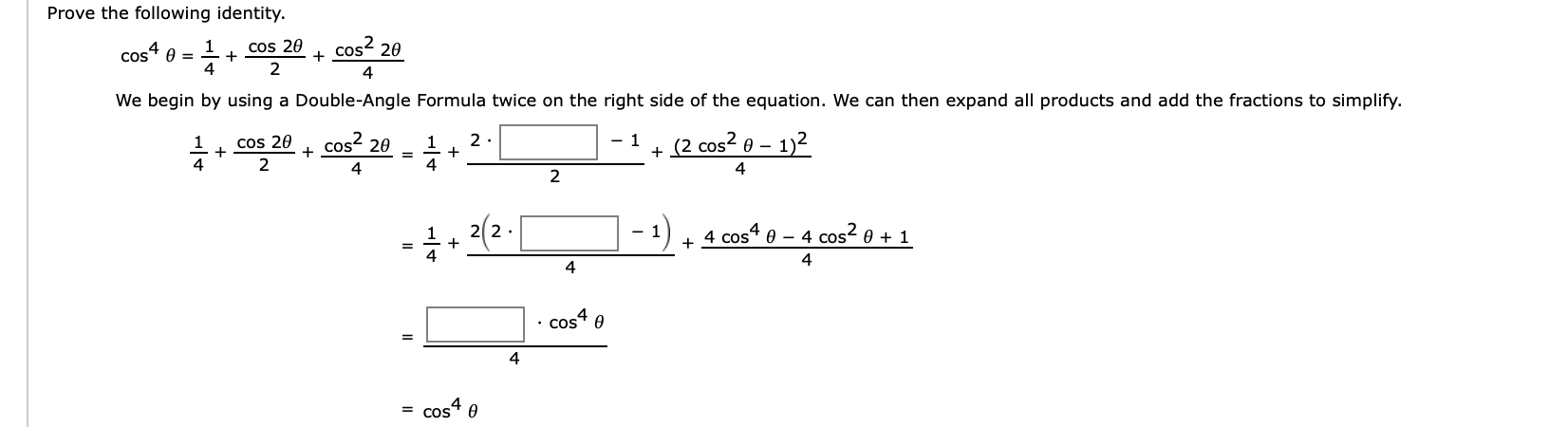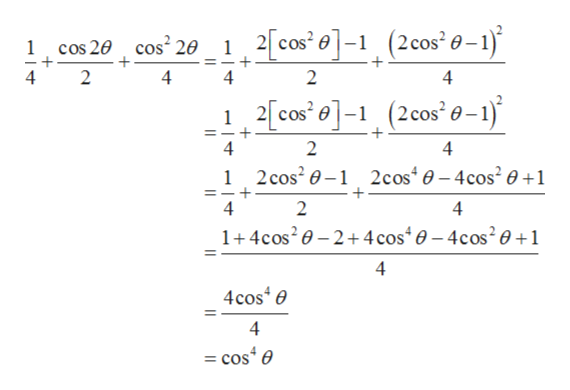# Prove the following identitycos2 201cos 20cos4=424We begin by using a Double-Angle Formula twice on the right side of the equation. We can then expand all products and add the fractions to simplify.cos2 20(2 cos2 02.1)cos 20+- 1+1142222.- 10 - 4 cos2 e + 14 cos444cos4 e= COS

Questionhelp_outlineImage TranscriptioncloseProve the following identity cos2 20 1 cos 20 cos4 = 4 2 4 We begin by using a Double-Angle Formula twice on the right side of the equation. We can then expand all products and add the fractions to simplify. cos2 20 (2 cos2 0 2. 1) cos 20 + - 1 + 1 1 4 2 2 22. - 1 0 - 4 cos2 e + 1 4 cos4 4 4 cos4 e = COS fullscreen
check_circleExpert Solution
Step 1

Given: -

Step 2

To find: -

Step 3

Calculation...help_outlineImage Transcriptionclose(2 cos' -1) 12cos0- cos2 20 cos 20 1 4 2 4 4 2 4 2 cos - (2cos' 0-1) =- 4 2 4 1 2cos20-1 2cos40-4cos2 0+1 4 2 4 1+4cos20-2+4 cos 0-4cos201 4 4cose 4 =cose fullscreen

### Want to see the full answer?

See Solution

#### Want to see this answer and more?

Solutions are written by subject experts who are available 24/7. Questions are typically answered within 1 hour*

See Solution
*Response times may vary by subject and question
Tagged in

### Trigonometry